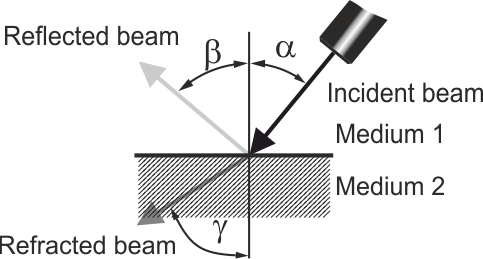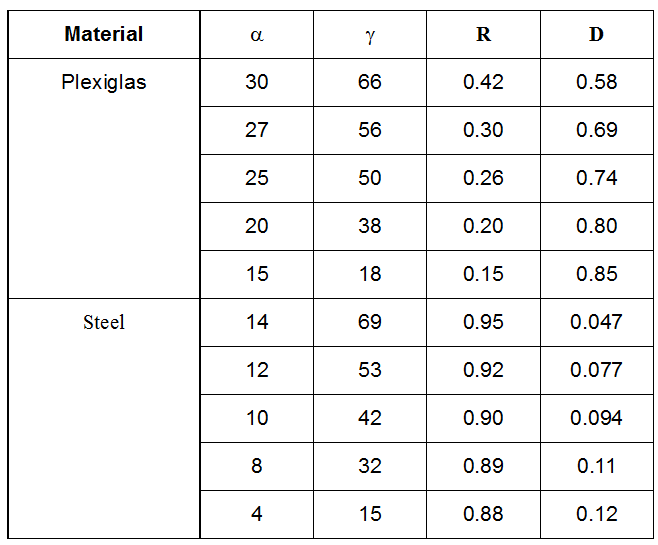# Intensity of the US field after crossing a wall

When an ultrasonic beam encounters a wall, a part of its energy is reflected and an other part is refracted. The intensity of both beams, the reflected and the refracted beams, can be computed when the two following parameters are known:

• the acoustic impedance of the medium
• the angle of incidence.The simple equations below assume a plane infinite wall or interface. This is of course never the case. Nevertheless, these three equations could really help because:

• they give an approximation of the total reflection angle.
• they predict the amount of ultrasonic energy that will penetrate in the liquid.
• they predict the amount of energy that tends to remain in the wall.
where:

Zi is the acoustic impedance of the medium i, which is equal to the product of the sound speed by the density of the medium i;

R is the ratio of the reflected intensity to the incident intensity;

D is the ratio of the refracted intensity to the incident intensity.

From these equations, it is possible to compute the value of the angle for which all the ultrasonic waves will be reflected. By setting a value of 90 degrees to the refracted angle, we can compute the value of the total reflection angle. For instance:

• for aluminum, its value is 14 degrees
• for steel, its values is 15 degrees
• for Plexiglas, its value is 33 degrees
We can also see the very strong influence of the wall material when the angle of incidence is different from the perpendicular. The two examples below show this influence very well.